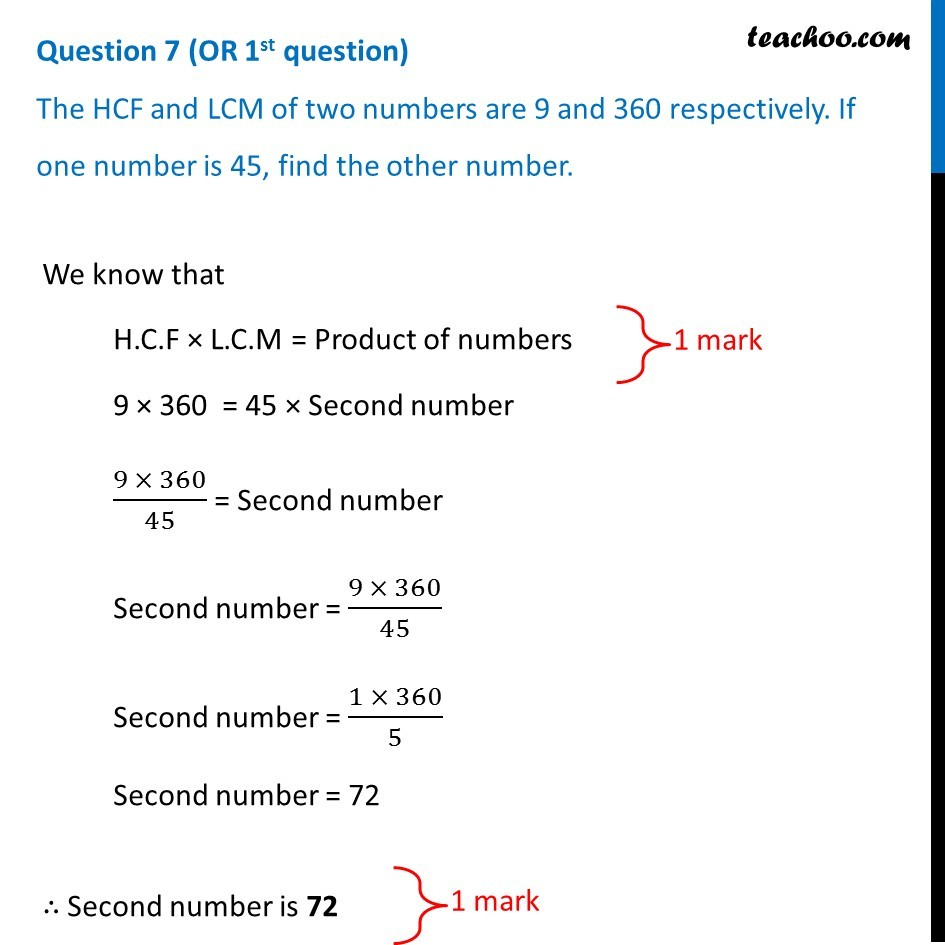CBSE Class 10 Sample Paper for 2019 Boards

Class 10
Solutions of Sample Papers for Class 10 Boards

Question 7 (OR 1 st question)

The HCF and LCM of two numbers are 9 and 360 respectively. If one number is 45, find the other number.Learn in your speed, with individual attention - Teachoo Maths 1-on-1 Class

### Transcript

Question 7 (OR 1st question) The HCF and LCM of two numbers are 9 and 360 respectively. If one number is 45, find the other number. We know that H.C.F × L.C.M = Product of numbers 9 × 360 = 45 × Second number (9 × 360)/45 = Second number Second number = (9 × 360)/45 Second number = (1 × 360)/5 Second number = 72 ∴ Second number is 72